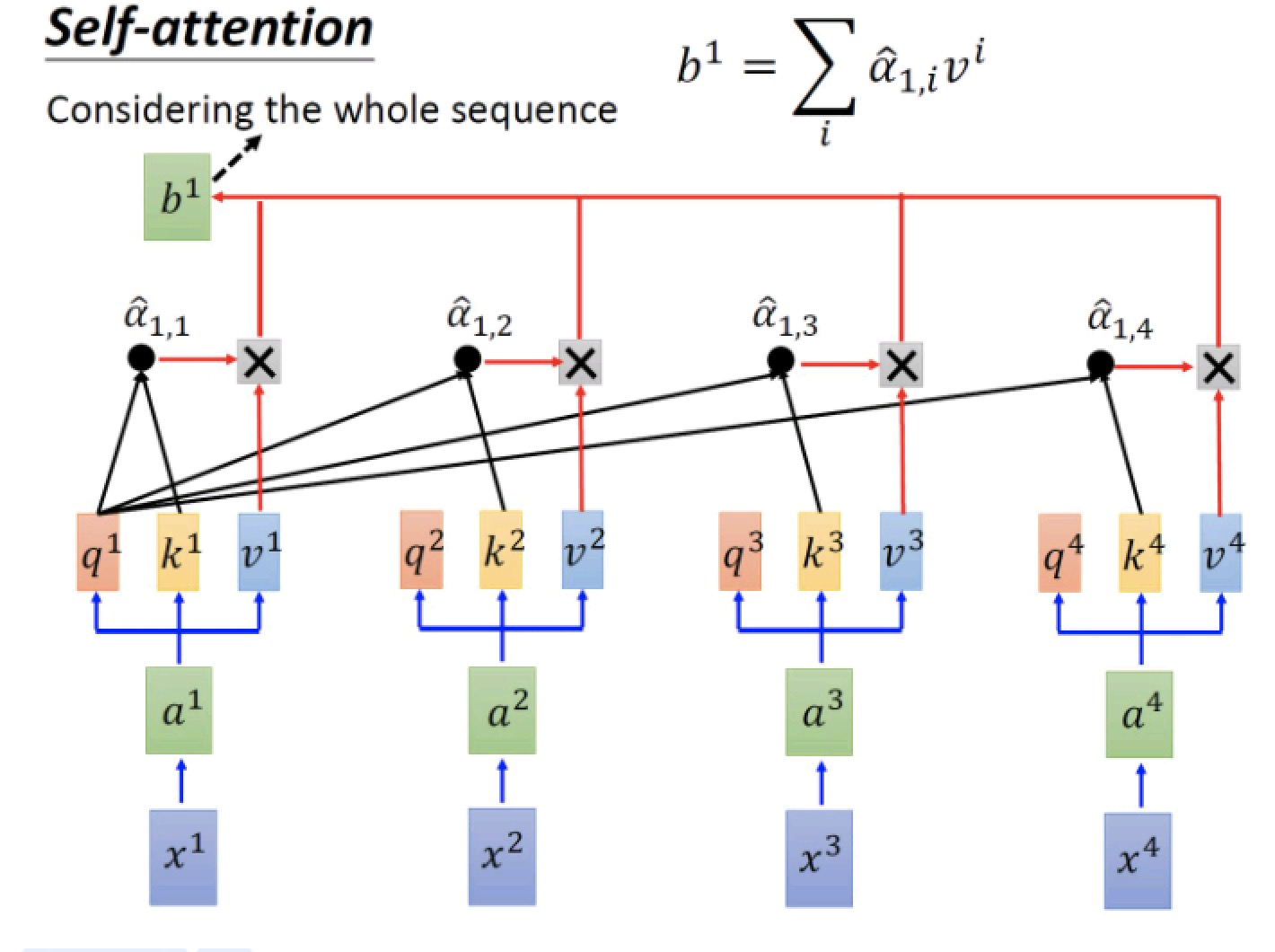# Transformer 个人小结

transformer 已经出来三年了，自己也没有深入了解，触及皮毛，最近想研究一下detr, 顺便写个 📒 记录一下对transformer的理解，个人主要从代码角度理解它的训练、测试的输入输出，关于理论网上太多了，自己只记录几个重点。这篇博客主要参考于李宏毅教授的课程以及 pytorch的transformer代码。

## self-attention## 位置编码def _get_sinusoid_encoding_table(self, n_position, d_hid):
def get_position_angle_vec(position):
return [position / np.power(10000, 2 * (hid_j // 2) / d_hid) for hid_j in range(d_hid)]
sinusoid_table = np.array([get_position_angle_vec(pos_i) for pos_i in range(n_position)])
sinusoid_table[:, 0::2] = np.sin(sinusoid_table[:, 0::2])
sinusoid_table[:, 1::2] = np.cos(sinusoid_table[:, 1::2])



## FFN

class PositionwiseFeedForward(nn.Module):
''' A two-feed-forward-layer module '''

def __init__(self, d_in, d_hid, dropout=0.1):
super().__init__()
self.w_1 = nn.Linear(d_in, d_hid) # position-wise
self.w_2 = nn.Linear(d_hid, d_in) # position-wise
self.layer_norm = nn.LayerNorm(d_in, eps=1e-6)
self.dropout = nn.Dropout(dropout)

def forward(self, x):

residual = x

x = self.w_2(F.relu(self.w_1(x)))
x = self.dropout(x)
x += residual

x = self.layer_norm(x)

return x



## transformer结构|true | false | false|
|true | true  | false|
|true | true  | true |


class ScaledDotProductAttention(nn.Module):
''' Scaled Dot-Product Attention '''

def __init__(self, temperature, attn_dropout=0.1):
super().__init__()
self.temperature = temperature
self.dropout = nn.Dropout(attn_dropout)

def forward(self, q, k, v, mask=None):

attn = torch.matmul(q / self.temperature, k.transpose(2, 3))